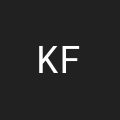Ben Nadel at the New York ColdFusion User Group (Jun. 2010) with: Andy Matthews ( @commadelimited ) Clark Valberg ( @clarkvalberg ) Ralf Korinth

By on

I am used to outputting a ColdFusion query horizontally (going across then breaking into another row). However, I need to display a query vertically through columns but I can't figure out how to loop over it or where to break the rows. Please help.

Looping over a query and outputting it across rows of table is nice and easy because there is no calculations that need to be done. Well not true, you do have to figure out when to break in to a new row, but that's a simple MOD calculation. If you want to display a query vertically, things get much more sticky because you are outputting in a horizontal methodology, but the cell value has to be based on the vertical position and column offset.

In order to figure out what query value to output you need to figure out where you are in terms of output row and column:

``````<!---
To demonstrate, first we have to build a test query.
For the purposes of demonstration, we are just going
to build a simple, number-incrementing query so you
can easily see where the values are output.
--->
<cfset qNumbers = QueryNew( "" ) />

<!--- Populate the query. --->
qNumbers,
"number",
"CF_SQL_VARCHAR",
ListToArray( "1,2,3,4,5,6,7,8,9,0" )
) />

<!---
In order for this to work, you have to explicitly
define how many columns you want to output.
--->
<cfset intColCount = 3 />

<!---
Based on the columns and the record count of the query,
we can determine how many rows we are going to output.
In this case, we are using the ceiling function as we
will need to round up.
--->
<cfset intRowCount = Ceiling(
qNumbers.RecordCount / intColCount
) />

<!---
Output the query. Unlike a traditional horizontally
outputted query, we cannot loop over the query itself
as it does not have "proper" ordering. Instead, we
have to loop over the rows and the columns and then
grab the appropriate value out of the query.
--->
<table>
<tr>

<!--- Loop over the rows, which we calculated above. --->
<cfloop
index="intRow"
from="1"
to="#intRowCount#">

<!--- Loop over the columns (set above). --->
<cfloop
index="intColumn"
from="1"
to="#intColCount#">

<!---
Here's where the magic happens. We need to
figure out which query cell value we are
getting based on the current row and column
indexes. If you think about it, the beginning
of each column begins with all the values
from the previous columns. That means that
each column begins with (COLUMN - 1) * the
number of rows in a column.
--->
<cfset intCellIndex = ((intRowCount * (intColumn - 1)) + intRow) />

<!---
Now, because we are outputting over a RxC
output, its possible that we will have a cell
that is outside of the record set. Check to
see if the cell index is beyond the record
count.
--->
<td>
<cfif (intCellIndex LTE qNumbers.RecordCount)>
#qNumbers[ "number" ][ intCellIndex ]#
<cfelse>
.<br />
</cfif>
</td>

</cfloop>

<!---
Check to see if we have any more rows to output.
Once we hit the final row, we will let the </tr>
post CFLoop handle the final close.
--->
<cfif (intRow LT intRowCount)>
</tr>
<tr>
</cfif>

</cfloop>

</tr>
</table>
``````

Running that gives us the following output:

[ 1 ][ 5 ][ 9 ]
[ 2 ][ 6 ][ 0 ]
[ 3 ][ 7 ][ . ]
[ 4 ][ 8 ][ . ]

It's a bit more work, but it gets the job done.

Want to use code from this post? Check out the license.It is producing an error:

Variable QNUMBERS is undefined.

The error occurred in D:\Sites\CF Test\index.cfm: line 14

12 : <body>
13 : <!--- To demonstrate, first we have to build a test query. For the purposes of demonstration, we are just going to build a simple, number-incrementing query so you can easily see where the values are output. --->
14 : <cfset QueryAddColumn(qNumbers,"number","CF_SQL_VARCHAR",ListToArray( "1,2,3,4,5,6,7,8,9,0" )) />@Ken,

Opps, I missed the very first line of code:

<cfset qNumbers = QueryNew( "" ) />

I have updated the code above. Sorry about that.I'm having trouble substituting a query in place of the number array you setup. I'm getting the right number of rows/columsn but as soon as I put the cfquery tag in, the output is wrong. I'm assuming I need to replace one of the cfloops with my cfoutput statements.DL, shoot me your code and I will take a look... ben [ at ] bennadel [ dot ] com.Thanks for the help, err. the code. I wasn't able to solve the problem but you sure did!

I look forward to seeing your posts in the other TAGS.Ben,
Would you mind posting the code that helped DL? I want to replace your array with a standard query.

--
Thanks for such a great blog!
Frank@Frank,

Glad you like the blog. The code above is actually working on a query. What you have to realize is that the query is being acted on just like it were an array. When I use the code:

#qNumbers[ "number" ][ intCellIndex ]#

That is access the query, "qNumbers", and getting the column value for the column "number" at row "intCellIndex".Ben,

Keep up the quality posts!

--
Frank